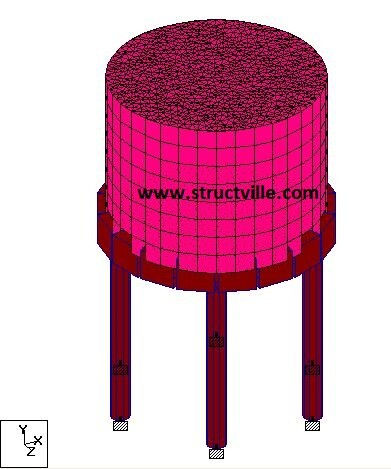# Effects of Temperature Difference on Cylindrical TanksIn our last post, we were able to evaluate the effect of temperature difference on rectangular tanks. In this article, we are going to evaluate the same effect on a cylindrical tank of the same volume, in order to obtain the internal stresses and displacements in the tank due to temperature difference. This post will serve as a comparison between the response of a rectangular and cylindrical tank to temperature actions.

In our last post, the dimensions of the rectangular tank was observed to be 3m (L) x 3m (B) x 2.5m (H), thereby giving a volume of 22.5 m3 . To model an equivalent cylindrical tank of the same height of 2.5 m, the diameter of the tank was obtained as 3.38 m. The other details of the tank are as follows;

Dimensions of columns = 300 mm diameter circular column
Dimension of beams = 300 x 500 mm
Height of column above ground level = 3 m
Diameter of tank = 3.38 m (centre to centre)
Height of tank = 2.5 m (centre to centre)
Thickness of tank walls and base = 250 mm
Support condition = Fixed
Temperature inside the tank = 120 oC
Temperature outside the tank = 25 oC
Maximum hydrostatic pressure from the liquid stored = 25 kPa
Modulus of elasticity of concrete = 2.8 x 107 kN/m2
Coefficient of expansion of concrete = 1.0 x 10-5 /oC

Temperature change for axial elongation = Average temperature = (25 + 120)/2 = 72.5 oC
Temperature difference = 25 – 120 = -95 oC

When modelled on Staad Pro using the procedure described in the video above, the configuration and results below were obtained.